# Fenchel-Moreau conjugate function

(diff) ← Older revision | Latest revision (diff) | Newer revision → (diff)

Given two sets,and a "coupling" function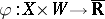, the Fenchel–Moreau conjugate to a function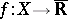with respect to the coupling functionis the functiondefined by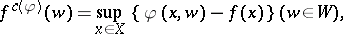(a1)

with the convention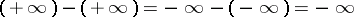[a1]. Whenandare linear spaces in duality, via a bilinear coupling function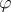(cf. also Linear space; Duality),is just the usual Fenchel conjugate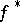(called also the Young–Fenchel conjugate, or Legendre–Fenchel conjugate; cf. also Legendre transform) of. Ifis a locally convex space and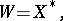the conjugate space of, with the coupling function, then the second Fenchel conjugateofcoincides with the greatest lower semi-continuous minorant of(Moreau's theorem); this result admits a natural extension to Fenchel–Moreau conjugates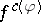.

Another important particular class of Fenchel–Moreau conjugates is obtained for coupling functionsthat take only the valuesand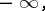or, equivalently, the conjugates for which there exists a (unique) subsetof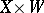such that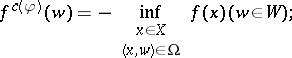(a2)

these are called conjugates of type Lau or level-set conjugates. While Fenchel conjugates have many applications in convex analysis, conjugates of type Lau are useful for the study of quasi-convex functions (i.e., of functions all of whose level sets are convex) and for duality theory in micro-economics (duality between direct and indirect utility functions).

A useful related concept is the Flachs–Pollatschek conjugate function, defined by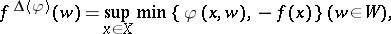(a3)

which has applications in, e.g., optimization theory.

A unified approach is the conjugate function with respect to a binary operation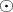on, assumed completely distributive (cf. also Completely distributive lattice) with respect to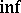in the lattice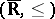, defined by(a4)

in particular, when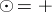(respectively,),is the Fenchel–Moreau (respectively, the Flachs–Pollatschek) conjugate function of.

In another direction, the Fenchel–Moreau conjugate has been generalized to functions with values in extensionsof ordered groups, with applications to functions in the extension (by adjoiningand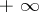) of the additive group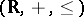and to functions in the extension (by adjoiningand) of the multiplicative group. More generally, one has also defined the conjugate function of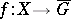with respect to a binary operationon, encompassing the preceding conjugates as particular cases.

One of the main fields of applications of these concepts is optimization theory: Whenis the objective function of an optimization problem, a conjugate function is used to define (the objective function of) a "dual" optimization problem.

For more details, see [a2], [a3], [a4].